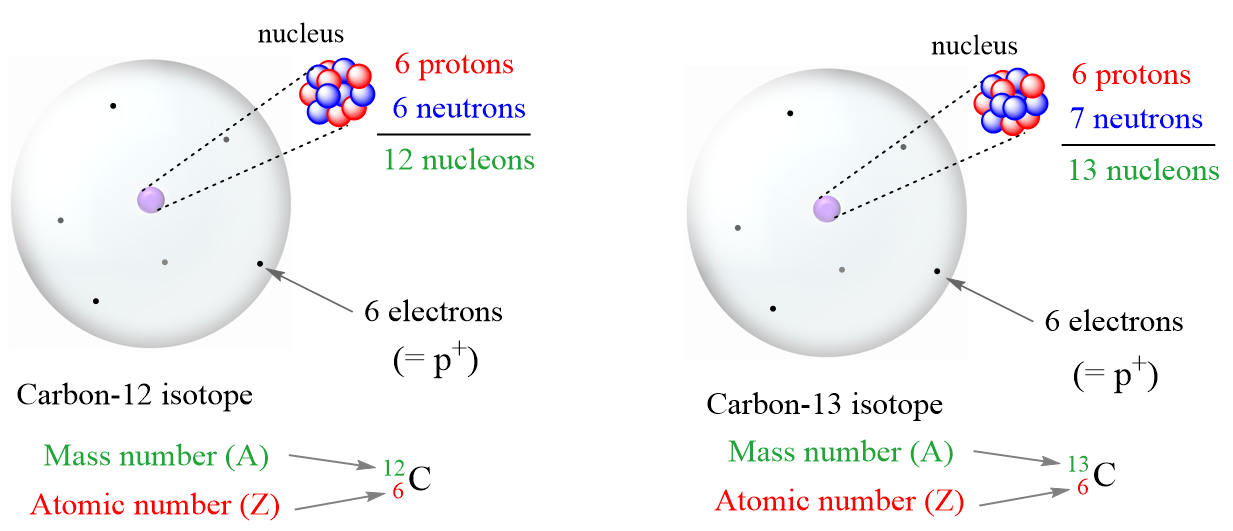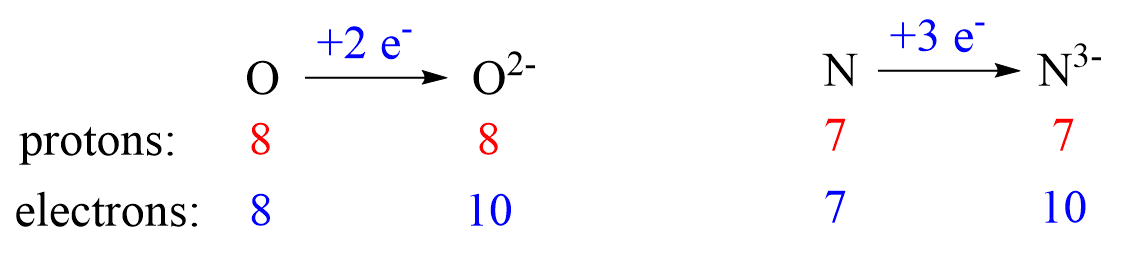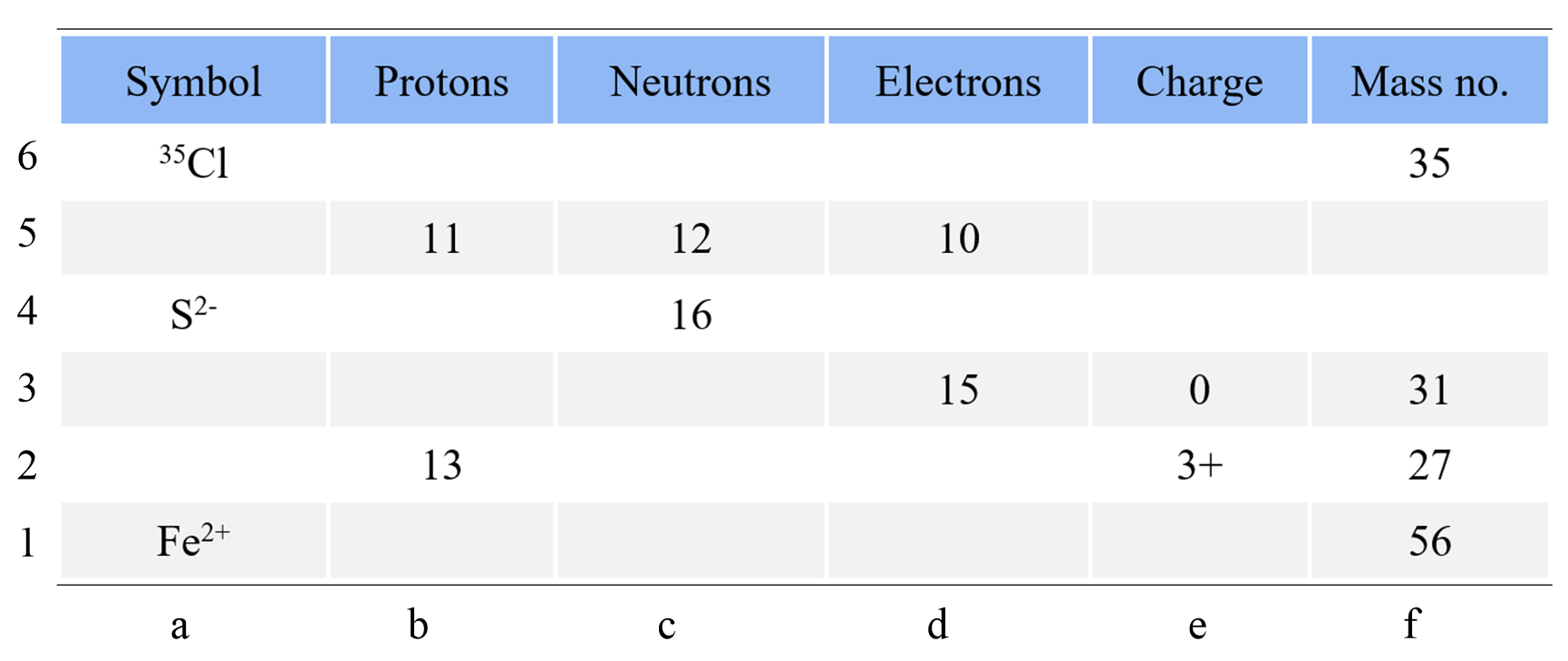## Atoms, Molecules, and Ions

Before talking about isotopes and subatomic particles, let’s first discuss the atomic structure. In a simplistic model, it is visualized as a sphere with a nucleus in the center and electrons (e) floating around it.Electrons are negatively charged subatomic particles. The charge is determined to be -1.6022 x 10-19 C (Coulomb). Now, most often you will see the charge of the electron as -1 and that is the relative charge which makes understanding concepts and comparing different particles easier. For example, protons, which together with neutrons make the nucleus of the atom, have the exact opposite charge of the electrons (+1.6022 x 10-19 C), and therefore, their relative charge is +1.

Neutrons (n), on the other hand, are electrically neutral particles slightly heavier than protons. A nucleon is a general term for both protons and neutrons. Both are extremely heavier than electrons and that is why the nucleus makes most of the atomic mass, even though it is very tiny compared to the size of the atom.

The masses of protons, neutrons, and electrons are:

m (e) = 9.10938 x 10-28 g

m (p) = 1.67262 x 10-24 g

m (n) = 1.67493 x 10-24 g

The number of protons is the atomic number (Z) of the element which we can find on the periodic table. The atomic number increases by one since every element has one more proton than the previous one in the periodic table. For example, the atomic number of boron is 5 because it has 5 protons, and the next element, carbon has 6 protons:The number of protons and electrons in an atom is always equal which makes them electrically neutral (no charge).

# Isotopes

The number of protons is always the same for a given element while the number of neutrons may vary and that’s how when we classify the atoms as isotopes:

Isotopes are atoms with the same number of protons but different numbers of neutrons. So, isotopes are different atoms of the same element. For example, compare the two isotopes of carbon:Let’s summarize some key observations of these isotopes:

• Both have the same number of protons.
• The number of neutrons is different.
• The mass number (A) is different because it is the sum of the number of protons and neutrons.
• The mass number is written in the upper-left corner of the symbol.
• The atomic number is written in the bottom-left corner of the symbol.

Notice also that the number of electrons is equal to the number of protons regardless of which isotope it is. This is true as long as we are talking about atoms.

For example, determine the number of protons, neutrons, and electrons in each of the following species:

$_{{\rm{11}}}^{{\rm{22}}}{\rm{Na,}}\;_{{\rm{26}}}^{{\rm{56}}}{\rm{Fe,}}\;_{{\rm{29}}}^{{\rm{65}}}{\rm{Cu}}$

• Na has 11 protons, and to find the number of neutrons, we subtract this number from its mass number:

n = 22 – 11 = 11

The number of electrons is equal to the number of protons, and it is also 11.

• Fe has 26 protons, and to find the number of neutrons, we subtract this number from its mass number:

n = 56 – 26 = 30

The number of electrons is equal to the number of protons, and it is 26.

• Cu has 29 protons, and to find the number of neutrons, we subtract this number from its mass number:

n = 65 – 29 = 36

The number of electrons is equal to the number of protons, and it is 29.

# Ions

Ions are charged particles derived from atoms by either a gain or a loss of an electron(s). It is this imbalance of positively charged protons and negatively charged electrons that makes ions charged particles. Whether an atom gains or loses electrons depends on the type of element it is.

Metals tend to lose electron(s) and become cations (positively charged ions).Nonmetals tend to gain an electron(s) and become anions (negatively charged ions).Notice that the number of protons is not changed, and the ions are charged because, unlike atoms, their number of protons and electrons are not equal.

The ionic charge is important to keep in mind when determining the number of protons, neutrons, electrons.

For example, determine the number of protons, neutrons, and electrons in each of the following species:

$_{}^{{\rm{32}}}{{\rm{S}}^{{\rm{2 – }}}}{\rm{,}}\;_{}^{{\rm{55}}}{\rm{M}}{{\rm{n}}^{{\rm{4 + }}}}$

• We find the number of protons for sulfur in the periodic table. The atomic number is 16 and that is the number of protons.

To find the number of neutrons, we subtract this number from its mass number:

n = 32 – 16 = 16

Because the charge is negative two, there are two more electrons than protons, so it is 16 + 2 = 18.

• We find the number of protons for Mn in the periodic table. The atomic number is 25 and that is the number of protons.

To find the number of neutrons, we subtract this number from its mass number:

n = 55 – 25 = 30

Because the charge is 4+, there are four more protons than electrons, so it is 25 – 4 = 21 electrons.

Check Also

#### Practice

1.

All the atoms of a given element have the same ________.

a) number of neutrons
b) number of protons
c) atomic mass
d) number of electrons
e) volume

This content is available to registered users only. Click here to Register! By joining Chemistry Steps, you will gain instant access to the answers and solutions for all the Practice Problems and the powerful set of General Chemistry 1 and 2 Summary Study Guides.
2.

Which atom has the smallest number of protons?

a) Li
b) Mn
c) Be
d) Ag
e) As

This content is available to registered users only. Click here to Register! By joining Chemistry Steps, you will gain instant access to the answers and solutions for all the Practice Problems and the powerful set of General Chemistry 1 and 2 Summary Study Guides.
3.

Which atom has the largest number of protons?

a) 11B
b) 14C
c) 14N
d) 19F
e) 18O

This content is available to registered users only. Click here to Register! By joining Chemistry Steps, you will gain instant access to the answers and solutions for all the Practice Problems and the powerful set of General Chemistry 1 and 2 Summary Study Guides.
4.

Which combination of protons, neutrons, and electrons is correct for the 59Ni isotope?

A) 29 p+, 30 n°, 29 e-
B) 28 p+, 30 n°, 28 e-
C) 28 p+, 31 n°, 28 e-
D) 28 p+, 32 n°, 31 e-
E) 27 p+, 32 n°, 27 e-

This content is available to registered users only. Click here to Register! By joining Chemistry Steps, you will gain instant access to the answers and solutions for all the Practice Problems and the powerful set of General Chemistry 1 and 2 Summary Study Guides.
5.

How many electrons are in the O2- ion?

a) 9
b) 10
c) 8
d) 11
e) 6

This content is available to registered users only. Click here to Register! By joining Chemistry Steps, you will gain instant access to the answers and solutions for all the Practice Problems and the powerful set of General Chemistry 1 and 2 Summary Study Guides.
6.

What is the average atomic mass of the element X if it contains 92.23 % of isotope X-27.976, 4.683 % of  X-28.976, and 3.087 % X-29.973?